# Speedy Rounding and Subtracting

With the help of these cute critters, your kid will be rounding off and subtracting numbers like a pro!### Rounding Numbers: Subtraction

As your second grader learns to round up or down and then complete subtraction equations, his overall maths skills will improve.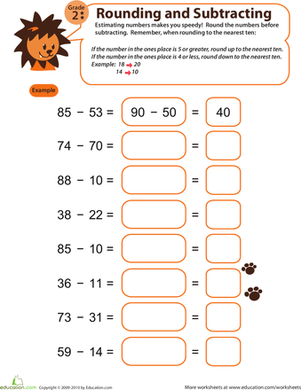### Speedy Rounding and Subtracting 2

Watch your year three's estimation and subtraction skills skyrocket with this fun rounding and subtracting worksheet!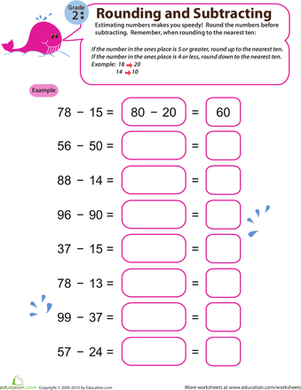### Speedy Rounding and Subtracting 3

With sufficient estimation practise, your second grader will gain a better understanding of numbers and significantly improve her subtraction skills!### Speedy Rounding and Subtracting 4

Sharpen your second grader's ability to subtract quickly and efficiently by integrating estimation into the process with this adorable worksheet.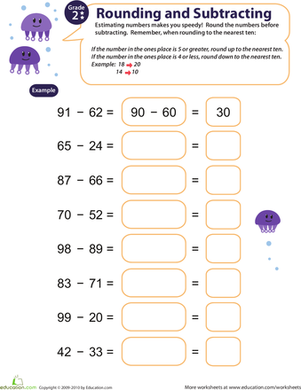### Speedy Rounding and Subtracting 5

With this adorable worksheet, your second grader will gain a better understanding of numbers and learn how to estimate subtraction problems quickly.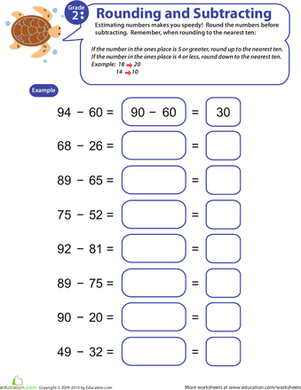### Speedy Rounding and Subtracting 6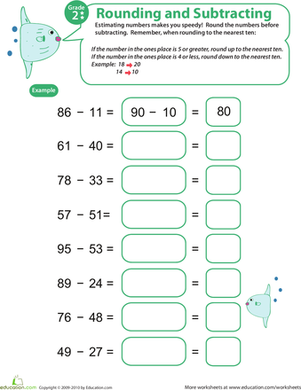### Speedy Rounding and Subtracting 7

Introduce your second grader to this cute worksheet, and watch her estimation and subtraction skills skyrocket!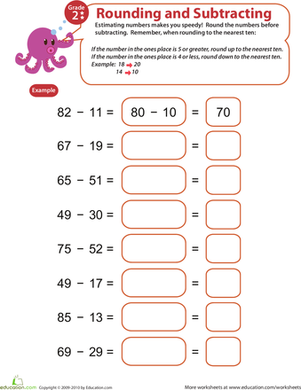### Speedy Rounding and Subtracting 8

We're willing to bet that your second grader will learn how to subtract quickly and efficiently by incorporating estimation into the process!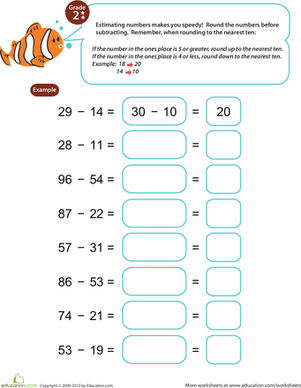### Speedy Rounding and Subtracting 9

As your second grader practices working with rounded numbers, he will improve not only his subtraction, but his overall maths skills as well.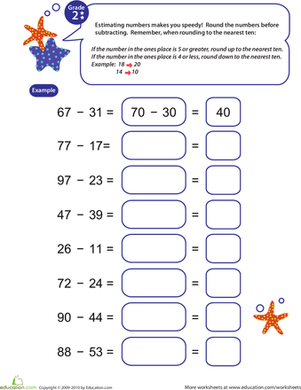### Speedy Rounding and Subtracting 10

Improve your second grader's subtraction and estimation skills with this charming, colorful worksheet.

Create new collection

0

### New Collection>

0Items

What could we do to improve Education.com?